#### You may also like### I'm Eight

Find a great variety of ways of asking questions which make 8.### Let's Investigate Triangles

Vincent and Tara are making triangles with the class construction set. They have a pile of strips of different lengths. How many different triangles can they make?### Noah

Noah saw 12 legs walk by into the Ark. How many creatures did he see?

# Break it Up!

## Break it Up!

You have a stick of $7$ interlocking cubes. You cannot change the order of the cubes.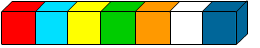You break off a bit of it leaving it in two pieces.

Here are $3$ of the ways in which you can do it: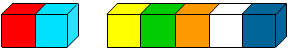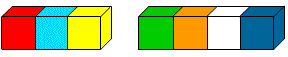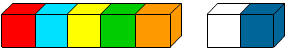In how many different ways can it be done?

Now try with a stick of $8$ cubes and a stick of $6$ cubes: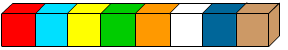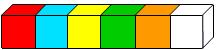Make a table of your results like this:

 Number of cubes Number of ways $6$ cubes ? $7$ cubes ? $8$ cubes ?

Now predict how many ways there will be with $5$ cubes.

Were you right?

How many ways with $20$ cubes? $50$ cubes? $100$ cubes?

ANY number of cubes?

* * * * * * * * * * * * * * * * * * * *

If all the cubes are the same colour, a split of $4$ and $2$ will look the same as a split of $2$ and $4$.

How many ways are there of splitting $6$ cubes now?

Can you predict how may ways there will be with any number of cubes?

### Why do this problem?

This problem makes a very good introduction to algebraic thinking, however, it also begins very simply so everyone can make a reasonable start.

### Possible approach

You could start by giving all the group some interlocking cubes and asking them to make a stick of seven. Then tell them to break their stick into two pieces and hold one piece in each hand. Ask how they have done this and write the various ways on the board and then enquire if there are any other ways that it could have been done. Is there a way that the results can be organised better? This may be the time to show the group how to make a simple table to record their results.

Some learners may claim that $2 + 5$ is the same as $5 + 2$. "Not always!" is the answer to this. Of course, numerically they are identical, but the context is also important. A good example for when this is not so is this. If five people are sitting down to a meal and two more turn up, it is quite possible there will be enough food for them. If however, two are starting their meal it is very unlikely that there will be enough food for five more! In this case, is $5 + 2$ the same as $2 + 5$? Just because the answer is the same, it does not mean that the question is the same!

After the introduction, learners could work in pairs on finding the number of ways that sticks of six, eight and nine cubes can be broken into two pieces. Then they should record their findings. Supply squared paper for those who wish to record using it.

When learners feel ready to generalise they can go on to working out the number of ways with $20$, $50$ and $100$ cubes and then ANY number of cubes.

At the end of the lesson all can come together to discuss their findings. They can be asked how they knew they had found all the ways of breaking a stick into two pieces. Those who know a good way of expressing "any number" can explain their reasoning.

### Key questions

How are you going to record what you have done?
How do you know you have found all the ways of breaking it into two pieces?
If you break it into $0 + 7$, does this give you two pieces?
How many ways do you think you can break $20/50/100$ cubes?
Can you see a connection between the total number of cubes and the number of ways you can break the stick into two pieces?
How could you express that "for any number"?

### Possible extension

Learners who can generalise the first part of the problem could then go on to exploring the second part - if all the cubes are the same colour, looking at the difference between odd and even numbers.

Then they could try a different problem in which generalisation is required such as Sticky Triangles.

### Possible support

Suggest working with the interlocking cubes and recording on squared paper.